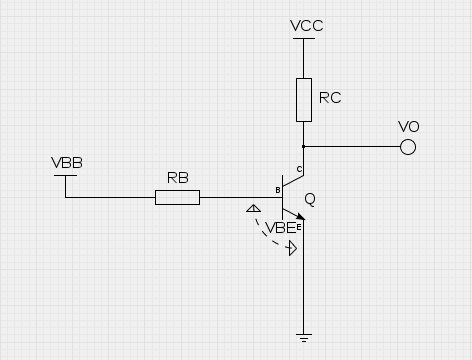OVERVIEW This is a design tool to calculate a suitable base resistor value for a switching application where a BJT is used to switch the output voltage voltage at the 'load' resistance RC between VCC and GND. This circuit can be used for example with a relay, when the relay coil DC resistance is known (measure it!). In that relay example application, the values could be: VCC=5.0, VBB=3.3, hFE=100, RC=100, which gives RB=4700. When VBB=3.3, the switch is open and VO is nearly GND. When VBB=GND, the switch is closed and VO is nearly VCC.Select the operating voltage VCC VCC: volts RESULTS Base resistor value: RB : ohms BJT Current gain factor from datasheet (use minimum value of hFE) hFE: const The base-emitter voltage, which is set to 0.7 V by default. VBE: volts Select the base switching voltage VBB VBB: volts Select the collector resistor RC RC: ohms